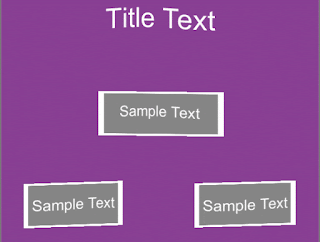A shader that will a add a bit of liveliness to your UI is always a good thing, especially when you are making a mobile game where grabbing and holding user attention is top priority.Jiggly Wiggly UI
This effect is known to hypnotize users into spending big bucks in your game (sarcasm). Anyway this shader is based on the optimized UI shader provided by Unity.
This optimized, mobile friendly UI shader can be found HERE.
Masking, Clipping and some other fancy stuff is not available when using this shader on UI components.
Let's get to making out jiggly wiggly UI shader.
We will look at the properties being declared :
``````Properties
{
[PerRendererData] _MainTex("Sprite Texture", 2D) = "white" {}
_Color("Tint", Color) = (1,1,1,1)
_WobblesPerSecond("Wobbles per Second ", Float) = 2
_WobblePeriod("Wobble Period (pixels)", Float) = 45
_WobbleAmplitude("Wobble Amplitude (pixels)", Float) = 3.2
}``````
Here are the properties defined in the CG PROGRAM along with #defines :
``````sampler2D _MainTex;
fixed4 _Color;

/*1*/float _WobblesPerSecond;
/*2*/float _WobblePeriod;
/*3*/float _WobbleAmplitude;
/*4*/#define TWO_PI 6.28318
}``````
The things added on top of the optimized UI shader are the three floats - (1), (2) & (3) and we also define the value of 2x PI (4) as we will be using it in the vertex shader.
Now take a look the vertex shader as it does all the work :
``````v2f vert(appdata_t IN)
{
v2f OUT;
/*1*/float4 worldPos = IN.vertex;
/*2*/float offsetInput = (_Time.y + frac(worldPos.x)) * _WobblesPerSecond * TWO_PI + worldPos.x / _WobblePeriod;
/*3*/worldPos.y += sin(offsetInput) * _WobbleAmplitude;
/*4*/worldPos.x += cos(offsetInput) * _WobbleAmplitude;
/*5*/OUT.vertex = UnityObjectToClipPos(worldPos);
OUT.texcoord = IN.texcoord;
#ifdef UNITY_HALF_TEXEL_OFFSET
OUT.vertex.xy += (_ScreenParams.zw - 1.0)*float2(-1,1);
#endif
OUT.color = IN.color * _Color;
return OUT;
}``````
Let's breakdown the steps:
1. We are storing world space position of vertex in 'worldPos'.
2. Calculate the offset that will be applied on the vertex position.
3. 'frac(worldPos.x)' acts as random seed value so that each objects wiggles differently and addition with '_Time.y' will keep changing the offset value every frame.
Then the '_WobblesPerSecond' multiplied with 'TWO_PI' increases the frequency of the wiggle effect. ' + worldPos.x / _WobblePeriod' adds additional variance to the wiggle movement, A larger 'WobblePeroid' means a larger distance between a crest and a trough and vise-versa.
4. Changing the y position of vertex with 'sin(offsetInput)' which gives -1 to 1 result and then multiplying with '_WobbleAmplitude' to get results in '-_WobbleAmplitude' to '+_WobbleAmplitude'.
5. Same thing as (3) except it's for changing x position of vertex and cosine is used.
6. Converting the vertices from world space to clip space which gives the output for the vertex shader.
The fragment shader does not undergo any changes. The default values present in the UI shader is enough.
Support Bitshift Programmer by leaving a like on Bitshift Programmer Facebook Page and be updated as soon as there is a new blog post.